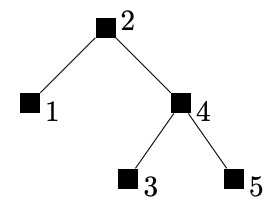시간 제한 메모리 제한 제출 정답 맞은 사람 정답 비율
8 초 256 MB 26 2 2 66.667%

## 문제

Johnny wrote a permutation to binary search trees (BST) converter: given a permutation $(\pi_1, \pi_2, \ldots, \pi_n)$ it assigns $\pi_1$ to the root of the BST, from numbers in $(\pi_2, \pi_3, \ldots, \pi_n)$ smaller than $\pi_1$ (in the same order!) it recursively creates a BST and attaches it as a left subtree of the root; symmetrically, from numbers in $(\pi_2, \pi_3, \ldots, \pi_n)$ larger than $\pi_1$ it also creates a BST and attaches it as a right subtree of th root.

To Johnny's surprise, it turns out that different permutations can result in the same BST -- for instance the permutations $(2, 3, 1)$ and $(2, 1, 3)$ result in the same BST. He found this fact astonishing and immediately defined Johnny's Numbers $J_k$: the $k$-th Johnny's Number is the smallest $n$ such that  there is a BST on $n$ nodes labelled with numbers $1, 2, \ldots, n$, that can be obtained from exactly $k$ different permutations of the numbers  $1, 2, \ldots, n$.

The investigation of Johnny's Numbers is difficult and their popularity is decreasing. Help Johnny out--compute Johnny's Number $J_k$ for the given $k$.

## 입력

The first line of input consists a single natural number $k$ ($1\le k \le 10^{11}$).

## 출력

In the first line of the input print a single positive integer:  $k$-th Johnny's Number $J_k$, assuming that it exists and it is at most $5\,000$. Second line of the input must contain $J_k$ integers --- lexicographically minimal generating  permutation between $k$ ones.

Otherwise, that is if $J_k$ does not exists or it is larger than $5\,000$ you should write the word "NIE" (Polish for 'no').

## 예제 입력 1

8


## 예제 출력 1

5
2 1 4 3 5


## 힌트

The tree having exactly eight generating permutations is shown below:All permutations generating that tree are: $(2, 1, 4, 3, 5)$, $(2, 1, 4, 5, 3)$, $(2, 4, 1, 3, 5)$, $(2, 4, 1, 5, 3)$, $(2, 4, 3, 1, 5)$, $(2, 4, 3, 5, 1)$, $(2, 4, 5, 1, 3)$, $(2, 4, 5, 3, 1)$.